# Rhombus problem

Perimeter of the rhombus is 204 dm and it area is 2244 dm2. Calculate the height of the rhombus.

Result

h =  44 dm

#### Solution:

$h = \dfrac{ 4 \cdot 2244}{ 204 } = 44 \ \text{dm}$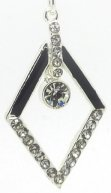Our examples were largely sent or created by pupils and students themselves. Therefore, we would be pleased if you could send us any errors you found, spelling mistakes, or rephasing the example. Thank you!

Leave us a comment of this math problem and its solution (i.e. if it is still somewhat unclear...):Be the first to comment!#### You need to know the following knowledge to solve this word math problem:

We encourage you to watch this tutorial video on this math problem:

## Next similar math problems:

1. Rhombus HP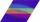Calculate area of the rhombus with height 24 dm and perimeter 12 dm.
2. Acreage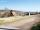Plot has a diamond shape, its side is 25.6 m long and the distance of the opposite sides is 22.2 meters. Calculate its acreage.
3. Strip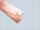From 5.9 cm wide strip should be cut rhombus with area 28 cm2. How long will be its side?
4. Write 3Write a real world problem involving the multiplication of a fraction and a whole number with a product that is between 8 and 10 then solve the problem
5. RectangleThe rectangle area is 182 dm2, its base is 14 dm. How long is the other side? Calculate its perimeter.
6. Odometer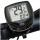The odometer is driven by rotation of the wheel whose diameter is 65 cm. After how many rotations the wheel turns counter to next kilometer?
7. The diameter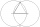The diameter of a circle is 4 feet. What is the circle's circumference?
8. Right triangleRight triangle legs has lengths 630 mm and 411 dm. Calculate the area of this triangle.
9. Fractions 3Calculate 1/9 of 27:
10. Two divided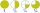Two divided by nine tenths.
11. Double 5Peter was thinking of a number. Peter doubles it and gets an answer of 8.6. What was the original number?
12. Pie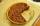Vili ate three pieces of pie . If it piece is 1/8 how much pie did he eat?
13. Pie IIVili ate three pieces of pie. If it pieces is 1/8 how much pie did he eat?
14. Fractions 4How many 2/3s are in 6?
15. Crates 2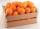One crate will hold 50 oranges. If Bob needs to ship 932 oranges, how many crates will he need?
16. A baker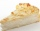A baker has 5 1/4 pies in her shop. She cut the pies in pieces that are each 1/8 of a whole pie. How many pieces of pie does she have?
17. A store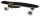A store received a shipment of 240 skateboards. In three weeks, it sold 1/3 of those skateboards. How many skateboards did the store sell?